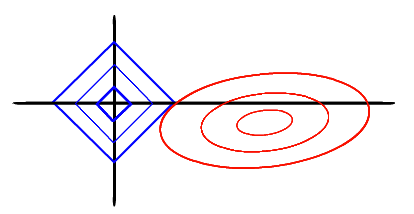# d2l自学笔记 – 4.多层感知机

《动手学深度学习》 — 动手学深度学习 2.0.0-beta0 documentation (d2l.ai)

# 多层感知机

## 基本概念

• 多层感知机（multilayer perceptron, MLP）
• 输入层 – 隐藏层（hidden layer） – 输出层
• 激活函数（activation function）

## 常见激活函数

### ReLU函数（Rectified linear unit, 修正线性单元）

$\operatorname{ReLU}(x)=\max (x, 0)$

### pReLU函数（Parametric Rectified Linear Unit, 参数化修正线性单元）

$\operatorname{pReLU}(x,\alpha)=\max (0,x)+\alpha\min(0,x)$

### sigmoid函数（又称squashing function, 挤压函数）

$\operatorname{sigmoid}(x)=\frac{1}{1+e^{-x}}$

### tanh函数（双曲正切函数）

$\operatorname{tanh}(x)=\frac{e^{x}-e^{-x}}{e^{x}+e^{-x}}=\frac{1-e^{-2x}}{1+e^{-2x}}$

## 模型与模型参数的初始化

num_inputs, num_outputs, num_hiddens = 784, 10, 256

W1 = nn.Parameter(torch.randn(
num_inputs, num_hiddens, requires_grad=True) * 0.01)
b1 = nn.Parameter(torch.zeros(num_hiddens,
W2 = nn.Parameter(torch.randn(
num_hiddens, num_outputs, requires_grad=True) * 0.01)
b2 = nn.Parameter(torch.zeros(num_outputs, requires_grad=True))

def relu(X):

def net(X):
X = X.reshape((-1, num_inputs))
H = relu([email protected] + b1)
return ([email protected] + b2)


net = nn.Sequential(nn.Flatten(),
nn.Linear(num_inputs, num_hiddens),
nn.ReLU(),
nn.Linear(num_hiddens, num_outputs)
)

def init_weights(m):
if type(m) == nn.Linear:
nn.init.normal_(m.weight, std=0.01)

net.apply(init_weights)


# 模型选择、欠拟合与过拟合

## 基本概念

• 模式（pattern）
目标：发现反应训练集潜在总体规律的模式$\rightarrow$泛化能力
• 欠拟合（underfitting）
• 过拟合（overfitting）
正则化（regularization）：用于对抗过拟合的技术
• 训练误差（training error）
• 泛化误差（generalization error）
• 训练集（train） – 验证集（validation） – 测试集（test）

## 影响模型泛化的因素

1. 可调整参数的数量
2. 参数采用的值（权重取值范围较大时，模型可能更容易过拟合）
3. 训练样本的数量

## 权重衰减（weight decay, $L_2$正则化）

1. 限制特征数量
2. 限制模型参数选择范围

$L(\mathbf{w}, b)+\frac{\lambda}{2}\|\mathbf{w}\|^{2} \\ \mathbf{w} \leftarrow(1-\eta \lambda) \mathbf{w}-\frac{\eta}{|\mathcal{B}|} \sum_{i \in B} \mathbf{x}^{(i)}\left(\mathbf{w}^{\top} \mathbf{x}^{(i)}+b-y^{(i)}\right)$

$\lambda$为正则化常数，是一个非负超参数。

### $L_2$正则化线性模型：岭回归（ridge regression）• 对权重向量的大分量施加了巨大的乘法
• 偏向于在大量特征上均匀分布权重的模型
• 可能使对单个变量的观测误差更稳定（为什么？

### $L_1$正则化线性模型：套索回归（lasso regression）• 权重集中于一小部分特征
• 其他权重清零（可以从图中蓝色方形与红色椭圆的交点看出）
• 具有特征选择（feature selection）的能力

### 权重衰减的实现

trainer = torch.optim.SGD([
{'params': net.weight, 'weight_decay': wd},
{'params': net.bias}], lr=lr)


## 暂退法（Dropout）

### 是什么？

$h^{\prime}= \begin{cases}0 & \text { 概率为 } p \\ \frac{h}{1-p} & \text { 其他情况 }\end{cases}$

### 实现

net = nn.Sequential(
nn.Linear(784, 256),
nn.ReLU(),
nn.Dropout(dropout1),
nn.Linear(256, 256),
nn.ReLU(),
nn.Dropout(dropout2),
nn.Linear(256, 10)
)


# 前向传播、反向传播和计算图

• 前向传播（forward propagation 或 forward pass）
• 矩阵的Frobenius范数：将矩阵展平为向量后应用的$L_2$范数
• 反向传播（backward propagation 或 backpropagation）

# 数值稳定性和模型初始化

$\partial_{\mathbf{W}^{(l)}} \mathbf{o}=\underbrace{\partial_{\mathbf{h}^{(L-1)}} \mathbf{h}^{(L)}}_{\mathbf{M}^{(L)} \stackrel{\text { def }}{=}} \cdot \ldots \cdot \underbrace{\partial_{\mathbf{h}^{(l)}} \mathbf{h}^{(l+1)}}_{\mathbf{M}^{(l+1) \text { def }}=} \underbrace{\partial_{\mathbf{W}^{(l)}} \mathbf{h}^{(l)}}_{\mathbf{v}^{(l)} \stackrel{\text { def }}{=}}$

• 参数更新过小，在每次更新时几乎不会移动，导致模型无法学习。
• 例如sigmoid函数，在输入很大或很小时，梯度都会消失。
相比之下，ReLU激活函数缓解了梯度消失问题，加速收敛。
• 网络较深时，容易在某一层切断梯度。

• 参数更新过大，破坏了模型的稳定收敛。

## 打破对称性

### Xavier参数初始化

$\frac{1}{2}\left(n_{\text {in }}+n_{\text {out }}\right) \sigma^{2}=1 \text { 或等价于 } \sigma=\sqrt{\frac{2}{n_{\text {in }}+n_{\text {out }}}}$

# 环境和分布偏移

## 经验风险与实际风险

### 经验风险（empirical risk）最小化

$\underset{f}{\operatorname{minimize}} \frac{1}{n} \sum_{i=1}^{n} l\left(f\left(\mathbf{x}_{i}\right), y_{i}\right)$

### 真实风险（true risk）

$E_{p(\mathbf{x}, y)}[l(f(\mathbf{x}), y)]=\iint l(f(\mathbf{x}), y) p(\mathbf{x}, y) d \mathbf{x} d y$

## 协变量偏移（covariate shift）

Covariate shift appears only in $X \rightarrow Y$ problems, and is defined as the case where $P_{\text {tra}}(y \mid x)=P_{\text{tst}}(y \mid x)$ and $P_{\text {tra}}(x)$ $\neq P_{\mathrm{tst}}(\mathrm{x})$

### 协变量偏移纠正

$\iint l(f(\mathbf{x}), y) p(y \mid \mathbf{x}) p(\mathbf{x}) d \mathbf{x} d y=\iint l(f(\mathbf{x}), y) q(y \mid \mathbf{x}) q(\mathbf{x}) \frac{p(\mathbf{x})}{q(\mathbf{x})} d \mathbf{x} d y$

$\underset{f}{\operatorname{minimize}} \frac{1}{n} \sum_{i=1}^{n} \beta_{i} l\left(f\left(\mathbf{x}_{i}\right), y_{i}\right)$

1. 生成一个二元分类数据集$\left\{\left(\mathbf{x}_{1},-1\right), \ldots,\left(\mathbf{x}_{n},-1\right),\left(\mathbf{u}_{1}, 1\right), \ldots,\left(\mathbf{u}_{m}, 1\right)\right\}$
2. 对数几率回归（logistic regression，softmax回归的二元分类特例） 训练二元分类器得到函数$h$
$P(z=1 \mid \mathbf{x})=\frac{p(\mathbf{x})}{p(\mathbf{x})+q(\mathbf{x})} \text { and hence } \frac{P(z=1 \mid \mathbf{x})}{P(z=-1 \mid \mathbf{x})}=\frac{p(\mathbf{x})}{q(\mathbf{x})}\\ P(z=1 \mid \mathbf{x})=\frac{1}{1+\exp (-h(\mathbf{x}))}$
3. 使用 $\beta_{i}=\exp \left(h\left(\mathbf{x}_{i}\right)\right)$ 或更好的 $\beta_{i}=\min \left(\exp \left(h\left(\mathbf{x}_{i}\right)\right), c\right)$ (c为常量) 对训练数据进行加权
4. 使用权重 $\beta_{i}$ 进行 $\left\{\left(\mathbf{x}_{1}, y_{1}\right), \ldots,\left(\mathbf{x}_{n}, y_{n}\right)\right\}$ 的训练

## 标签偏移（label shift, prior probability shift）

Prior probability shift appears only in $Y \rightarrow X$ problems, and is defined as the case where $P_{\text {tra}}(x \mid y)=P_{\text {tst}}(x \mid y)$ and $P_{\text {tra}}(y) \neq P_{\text{tst}}(y)$

### 标签偏移纠正

1. 计算验证集（为什么？）的混淆矩阵$\mathbf{C}$。该矩阵中每个单元格$c_{ij}$的值表示验证集中真实标签为$j$，模型预测为$i$的样本占比。

2. 将所有模型在测试时的预测取平均数，得到平均模型输出$\mu(\hat{\mathbf{y}}) \in \mathbb{R}^{k}$

3. 可以通过求解一个简单线性系统来估算测试集的标签分布$p$
$\mathbf{C} p(\mathbf{y})=\mu(\hat{\mathbf{y}})$
$\sum_{j=1}^{k} c_{i j} p\left(y_{j}\right)=\mu\left(\hat{y}_{i}\right)$

## 概念偏移（concept shift）

Concept shift is defined as

• $P_{\text {tra}}(y \mid x) \neq P_{\text{tst}}(y \mid x)$ and $P_{\text {tra}}(x)=P_{\text{tst}}(x)$ in $X \rightarrow Y$ problems.
• $P_{\text {tra}}(x \mid y) \neq P_{\text{tst}}(x \mid y)$ and $P_{\text{tra}}(y)=P_{tst}(y)$ in $Y \rightarrow X$ problems.

# 一些实战经验

## 对数差异

$e^{-\delta} \leq \frac{\hat{y}}{y} \leq e^{\delta}\Leftrightarrow |\log y-\log \hat{y}| \leq \delta$

$\sqrt{\frac{1}{n} \sum_{i=1}^{n}\left(\log y_{i}-\log \hat{y}_{i}\right)^{2}}$